# Hydrodynamics, mathematical problems in

Problems for systems of equations describing mechanical models of a liquid flow and its interaction with the bounding surfaces. The frequently-encountered turbulent flows are theoretically described with the aid of partial, and mostly semi-empirical, models that are applicable to rather narrow types of flow. In theoretical studies on liquid flow in which turbulence may be neglected, the model of a homogeneous incompressible liquid is extensively employed. This model is described by the Navier–Stokes equations: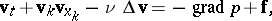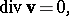where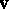is the velocity vector,is the pressure,is the external force vector acting on a unit mass, andis the coefficient of kinematic viscosity of the liquid, the density of which is taken to be one. If the coefficientis a function of the temperature, then the thermal-conductance equation in a moving medium, which allows for the production of heat as a result of viscosity-induced dissipation of mechanical energy, and which follows from the law of conservation of energy, is added to the Navier–Stokes equations.

The different problems in fluid mechanics yield different systems of additional conditions (initial conditions, boundary conditions) which must be imposed on the solution of the Navier–Stokes equations. Since the resulting mathematical problems in fluid mechanics are often complicated, simpler mechanical models are constructed. In problems of any particular class it is attempted to isolate the main factors on which the motion may depend, and only the terms allowing for these factors are preserved in the equations and in the additional conditions. In more complicated cases there may be different fundamental determining factors in different parts of the domain in which the motion takes place. The description of the motion as a whole then becomes a combination of solutions of local problems, which are solved with the aid of appropriate local algorithms.

The model of an ideal liquid — i.e. a model in which the effect of viscosity is neglected (i.e.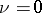) — has proved very useful in solving many types of problems.

In the presence of potential external forces(e.g. allowing for the force of gravity alone), a special role is played by potential flows of an ideal liquid for which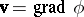in view of the fact that these are conserved. The potentialin such cases satisfies the Laplace equation:.

In this approximation, many problems in fluid mechanics are reduced to classical problems in potential theory. Thus, the problem of the motion of a solid body in a liquid at rest at infinity is reduced to solving an exterior Neumann problem. However, it is only in few cases that this solution leads to an approximate description of the velocity and of the fields of pressure in a real liquid. One such important case is the planar motion of a streamlined profile, with a constant circulation velocity around it.

In a viscous liquid the action of the viscosity in a boundary layer near the surface of the solid body produces a vorticity, so that a shear wake is generated behind the solid body. In the case of streamlined bodies (e.g. wings with a sharp rear edge, moving at small incidence angles), this wake is very thin if the Reynolds number is large and, when a model involving an ideal liquid is considered, it may be replaced by a discontinuity surface of the potential (a vortex layer). Thus there arises the problem of finding, outside the wing, the velocity potential with a discontinuity on the surface descending from the rear edge of the wing, the position of which is not known in advance, and which is determined by solving the problem. Analytical solutions of this problem were obtained only in a linear approximation for thin wings of simple form in the plane (a circle, an ellipse). Numerical methods for solving this problem are available for wings of other shapes for stationary and non-stationary motions. Numerical methods for computing the flow around wings resulting from non-linear flow disturbances are being developed.

A wide class of problems in hydrodynamics gives rise to problems concerning the determination of the potential motion of an ideal liquid in a domain partly bounded by solid walls and partly by a free surface. The shape of the free surface is not known in advance, but must be found in the course of solving the problem by imposing additional conditions on the surface. If it is permissible to neglect the effects of forces of gravity and surface tension, it follows from Bernoulli's integral in case of a stationary motion that the velocity of the liquid along the free surface, which is a contact surface with a domain of constant pressure, is constant. Typical problems of this type include the outflow of a jet of liquid from an opening in a vessel, and the mutual impact of liquid jets, flow of a liquid jet of constant thickness onto a solid body, and high-speed gliding motion of a solid body on the surface of a liquid. They also include problems of the unlimited flow of liquid around a solid body with a discontinuity in the stream, and the formation of stagnant zones, or cavities, with a constant pressure behind the body. All these problems are solved in the case of a planar flow by techniques of conformal mapping, variational methods and methods of integral equations. The solution of three-dimensional problems is much more difficult and is based on numerical methods.

The Cauchy–Lagrange integral yields a non-linear condition for the velocity potential on a free surface for the case of a non-stationary flow with free surfaces, with allowance of the effect of forces of gravity and of capillary forces. This condition is responsible for the main difficulties involved in solving such problems — the entry of a solid body into a liquid or its emergence from the surface of a liquid; gliding motion of a solid on the surface of a heavy liquid; the motion of a body partly or totally immersed in a heavy liquid; and waves on the surface of a heavy liquid.

Only a few results of solving problems of this kind have been obtained by exact methods. Approximate asymptotic methods were much more successful. One of the main approaches is the linear theory of small perturbations and its modifications aimed at increasing its rigour. The mathematical apparatus used for this purpose comprises almost the entire linear apparatus of mathematical physics.

The "shallow water" approximation is of importance in the study of waves on the surface of a heavy liquid; the meaning of it is that the depth of the liquid in the basin is small in comparison to the length of the waves under study. A non-linear theory of waves with a finite amplitude can be developed by means of this approximation. The theory can be reduced to a set of hyperbolic partial differential equations similar to the one arising in the theory of two-dimensional supersonic gas flow; many important results are obtainable by the method of characteristics. In the quasi-one-dimensional approximation the theory of shallow water forms the base of the branches of modern hydraulics dealing with wave motion in rivers and in long open channels; the propagation of flood waves and of waves generated by the destruction of dams; generation of periodic stationary waves in channels with a steep bottom; etc.

In the exact non-linear theory of waves on the surface of a heavy liquid methods of the theory of functions and functional analysis have made it possible to solve many existence and uniqueness problems of wave motions. In particular, the existence of smooth two-dimensional periodic progressive waves with a finite amplitude on the surface of a liquid of an infinite or a constant finite depth was proved.

The scheme of a potential stream flowing around a solid body with a discontinuity in the stream and with the formation of a stagnant domain in which the velocity of the liquid is zero is only one of the many possible schematizations. Schemes are also available for flows around a solid body with a domain in the wake filled with rotational flow. In the context of such schemes, and also in connection with several other applications, there arose the problem of matching the domains of a potential and a vortex flow, the shape of the interface between the two being unknown. Certain partial numerical solutions of this problem are available for a constant vorticity in the domain of vortex flow.

In the study of motion of liquids in which viscosity is a significant factor, a number of mathematical models — representing simplifications of the complete Navier–Stokes equations — were also developed. The most important and most extensively employed ones include the theory of slow Stokes motion and its strengthenings; the theory of the Prandtl boundary layer and its strengthenings; and the Boussinesq theory of thermal convective motion.

In a number of cases of liquid motion of practical interest, the force of inertia acting on an element of the liquid is small in comparison to the forces of pressure or viscosity (if similarity criteria are employed, this corresponds to small Reynolds numbers), so that it can be neglected to a first approximation (Stokes' theory). As a result arises the problem of determiningandin a liquid-filled space from the system of linear equations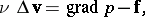This system of equations served to solve numerous problems on the motion of a viscous liquid in rigid and in deformable tubes and in gaps of various shapes between the fixed and mobile surfaces, as well as the problem of the motion of solid bodies and of gas bubbles in a viscous liquid. These problems form the base of the hydrodynamic theory of lubrication, and are also of importance in chemical technology and in biological and other applications.

Advances have been made on rigorously proving the solvability of the Stokes equations under various additional conditions. In particular, the solution has been found to the problem of flow around a system of bodies of finite sizes by an unlimited stream at a given velocity at infinity, and the uniqueness of the solution was demonstrated.

For planar flows the Stokes equations are reduced to the biharmonic equation with respect to the stream function. The boundary condition of the flow around a given contour leads to specifying the stream function itself and its normal derivative on the contour, so that the solution of the problem of flow around a solid in this case yields a problem in mathematical physics which has been thoroughly studied.

As distinct from a spatial flow around a solid, in the case of a planar flow (such as a cross flow around a circular cylinder) there is no solution of the problem for a given velocity of the flow at infinity. The "Stokes paradox" , and subsequently also the "Whitehead paradox" (it is impossible to find by iteration a successive approximation to the Reynolds number in the problem of flow around a sphere), stimulated the development in hydrodynamics of asymptotic methods for small Reynolds numbers, strengthening the theory of Stokes. These methods of matched asymptotic expansions found use not only in the problem of flow around a solid by a viscous fluid with a small Reynolds number, but also in many other problems in fluid mechanics. As applied to flow around solid bodies, the principle of the method may be stated as follows. The Stokes approximation is considered as the principal term of the asymptotic expansion for small Reynolds numbers Re in a neighbourhood of the solid body around which the flow is taking place. At larger distancesfrom the body (of the order of 1/Re) this expansion becomes unsuitable. For this reason, at some distance from the solid body, a contracted radial coordinateis introduced and another asymptotic expansion is constructed as a disturbance of the steady flow (Oseen expansion). The arbitrariness involved in expansions constructed in this way is eliminated by "matching" them in an intermediate domain by a procedure specially developed for this purpose.

Problems on flow around solid bodies are successfully solved for small Reynolds numbers by numerical methods. The results of asymptotic computations in the problem of flow around a sphere show a satisfactory agreement with the experimental values up to Reynolds numbers of about 60, which is close to the limit at which in experiments a stationary flow is observed.

Many cases of liquid motion through a porous medium are motions in which the inertial forces are small as compared to the viscous forces. It may be assumed that the flow of a liquid across a surface element intersecting a sufficiently large number of pores is proportional to the gradient of the pressure and inversely proportional to the viscosity coefficient; this is similar to the case in which the medium consists of a number of tubes of small diameter. If the structure of the medium is statistically isotropic, one obtains(Darcy's law). If the permeability coefficientof the medium is independent of the coordinates and the viscosity coefficientof the liquid is constant, then the velocity will be a potential vector, and the potential will satisfy the Laplace equation. Accordingly, potential theory can be successfully applied to the solution of various practical problems concerning the filtration of water under dams, the motion of ground water near coastal regions under the forces of ebb and tide, filtration of oil into oil wells, etc.

The solutions obtained for the problems of a continuous potential flow around bodies by an unlimited stream of an incompressible ideal liquid also satisfy the complete set of Navier–Stokes equations, but do not satisfy the conditions of adhesion of the liquid to the surface of the solid body. If the Reynolds number is large, the liquid layer near the surface of the solid body having a significant viscosity, owing to which the conditions of adhesion of the liquid to the solid body are satisfied, is thin. By making a comparative estimate of the order of magnitude of the various terms in the Navier–Stokes equations based on this thinness, the equations can be reduced to a simpler form — to the equations of boundary-layer theory (the Prandtl equations, cf. Prandtl equation). In the Prandtl equations the pressure remains unchanged along the normal to the surface of the solid body around which the flow takes place, while the variation of the pressure along the surface is determined by the flow of an ideal liquid outside the boundary layer. In the simplest case of a two-dimensional stationary motion around a plane contour, the Prandtl equations take the form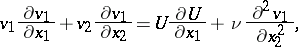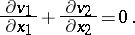Here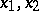and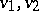are, respectively, the coordinates and velocity components in the boundary layer along the surface and along the normal to the surface, whileis the velocity on the external flow.

The boundary-layer equations yield the principal term in a suitably constructed asymptotic expansion of the Navier–Stokes equations for large Reynolds numbers.

The boundary-layer equations are of parabolic type with a line of degeneration on the contour of the solid body (where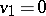). Various effective approximate methods have been developed for the integration of the boundary-layer equations in order to obtain characteristics of the flow inside it which are of importance in various applications; these include, in particular, methods of integral relations based on the use of various approximations for the distribution of the longitudinal velocityin the perpendicular direction inside the layer. Numerical methods yield results of practical importance relatively easy, including those applicable to three-dimensional flows, which are obtained by direct integration of the boundary-layer equations.

The general theory (i.e. the theory of existence and uniqueness) has been developed for the cases where inside the boundary layer on each normal to the surface around which the flow takes place the projection of the liquid velocity on the direction of the velocity of the external stream does not change sign (i.e. there are no "recirculating flows" ).

Perfectioning the theory of boundary layers by computing the successive terms of the asymptotic expansion for large Reynolds numbers involves the method of "matched" asymptotic expansions. For instance, the resistance coefficient of a plate could be determined with accuracy of order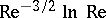(the order of the principal term is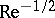).

In problems of thermal convection the liquid motion takes place in a field of potential mass forces, and is the result of different densities of different portions of the liquid, caused by its non-uniform heating. In the Boussinesq approximation convective motions are studied with the aid of a model of a viscous liquid of constant density, the equation of motion of which allows for the excessive mass force due to inhomogeneous temperatures: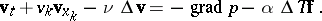Hereis the excess pressure over the hydrostatic pressure,is the excess of the temperature over its value corresponding to the unperturbed density andis the coefficient of the relative volume expansion of the liquid during its heating. This equation is supplemented by the equation of conservation of mass and by the heat equation in a moving medium (in both cases for a liquid of constant density; viscous dissipation is usually neglected in the heat equation).

Certain problems in convective motion are studied with the aid of the complete system of Navier–Stokes equations and are solved by numerical methods. Prior to the introduction of electronic computers, problems in fluid mechanics could not be solved by the use of the complete set of Navier–Stokes equations unless the non-linear terms of these equations vanished identically as a result of the special conditions inherent in the problem. A number of numerical algorithms are available for solving the Navier–Stokes equations; these are applicable for computing flows at small Reynolds numbers. The difficulties involved in the realization of numerical algorithms at large Reynolds numbers reflect the very properties of the solutions of the Navier–Stokes equations under these conditions. For instance, it was proved that solutions of stationary boundary value problems exist at all Reynolds numbers if the total flux of the liquid across each isolated part of the domain which is filled with the liquid is equal to zero. If this domain extends to infinity, the additional condition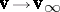is imposed at infinity. For a bounded domain and small Reynolds numbers the solution of the boundary value problem is unique and stable. If the Reynolds number becomes larger, this unique solution is no longer stable, and a new stationary stable solution appears. Such change in the flow may occur several times as Re keeps increasing.

It was proved for the general three-dimensional case that the initial or boundary value problems are uniquely solvable in a neighbourhood of smooth initial data; the problem of finding a unique solution of such problems in the large — that is, for any period of time and irrespective of the size of the domain of the motion — has not yet (1977) been solved. It would appear that the solutions of the non-stationary problem may become less and less regular in the course of time, may become branched and may pass into "turbulence" regimes.